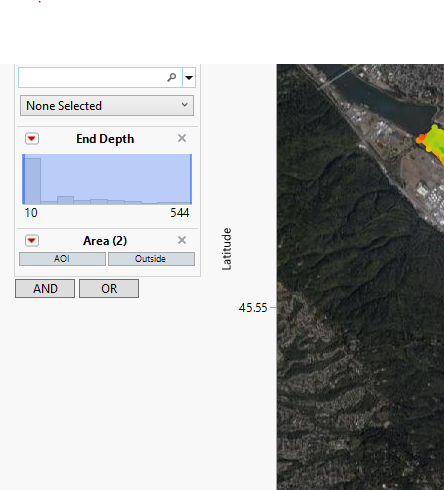Our World Statistics Day conversations have been a great reminder of how much statistics can inform our lives. Do you have an example of how statistics has made a difference in your life? Share your story with the Community!
Choose Language Hide Translation Bar
Highlighted

## Appending Slider to Graph Builder Contour Map Script

Hello,

I've written a script where I was able to append a slider for sampling depth onto a graph builder contour. However, the map is not responding and changing when the slider is being utilized.

I believe everything is referenced properly and I haven't got any errors with the log, but nothing is changing when I move the slider.

Script is attached.

``````Names Default to Here(1);
dt = Currentdatatable();

datacolumn=column(dt, "End Depth");
new window ("RFIS Contour Plot Depth Filtering",
window:gb = Graph Builder(
Size( 599, 514 ),
Show Control Panel( 0 ),
Variables(
X( :Longitude ),
Y( :Latitude ),
Color(
Transform Column( "Log[SUM TOC NORM]", Formula( Log( :SUM TOC NORM ) ) )
)
),
Elements(
Contour(
X,
Y,
Legend( 5 ),
Line( 1 ),
Boundary( 0 ),
Number of Levels( 10 ),
Alpha( 95.1827841988282 ),
Smoothness( 0.01 )
),
Points( X, Y, Legend( 6 ) )
),
Local Data Filter(
columns( :Sample Code, :Area ),
Where( :Area == "AOI" ),
Display(
:Sample Code,
"Single Category Display",
Find( Set Text( "" ) )
)
)
),
SendToReport(
Dispatch(
{},
"Longitude",
ScaleBox,
{Scale( "Geodesic" ), Format( "Best", 15 ), Min( -122.777380897341 ),
Max( -122.674219621177 ), Inc( 0.025 ), Minor Ticks( 0 )}
),
Dispatch(
{},
"Latitude",
ScaleBox,
{Scale( "Geodesic" ), Format( "Fixed Dec", 15, 2 ),
Min( 45.5156964729219 ), Max( 45.6018236979199 ), Inc( 0.05 ),
Minor Ticks( 0 )}
),
Dispatch(
{},
"400",
ScaleBox,
{Legend Model(
5,
Properties(
0,
{Color Theme(
{"Green Yellow Red Copy", 16387, {"Green", "Yellow",
{252, 11, 11}}, {0.273504273504274, 0.5,
0.789173789173789}}
), Width( 12 )}
)},
Item ID( "Log[SUM TOC NORM]", 1 )
)
), Legend Model(
6,
Properties(
0,
{Color Theme(
{"Green Yellow Red Copy", 16387, {"Green", "Yellow",
{252, 11, 11}}, {0.273504273504274, 0.5,
0.789173789173789}}
), Width( 12 ), N Labels( 10 )}
)},
Item ID( "Log[SUM TOC NORM]", 1 )
),
Properties( 1, {Marker Size( 4 )}, Item ID( "Latitude", 1 ) )
)}
),
Dispatch(
{},
"graph title",
TextEditBox,
{Set Text( "RFIS Contour Plot Depth Filtering" )}
),
Dispatch(
{},
"Graph Builder",
FrameBox,
{Background Map(
Images(
"Web Map Service",
"http://wms2.mapsavvy.com/WMSService.svc/f8e454cf75bf41a882e5126809b56a45/WMSLatLon",
"Aerial"
)
), Grid Line Order( 2 ), Reference Line Order( 3 )}
),
Dispatch(
{},
"400",
LegendBox,
{Legend Position( {5, , 6, [-1, -1]} ), Position( {0, -1, -1} )}
)
),
),
H List Box(
Text Box("Depth"),
window:slmin = col min(datacolumn);
window:slmax = col max(data column);
window:slider1 = SliderBox(window:slmin, window:slmax, window:slmax,
scale=Matrix( {slmin,slmax} );
scale=scale`;
window:gb <<SendToReport(
Dispatch({},"400",
ScaleBox,
{Legend Model( 3,
Properties(0,{gradient( {Scale Values( scale ), Width( 12 )} )})
)}
)
);
);

)
);``````

Thanks

4 REPLIES 4
Highlighted

## Re: Appending Slider to Graph Builder Contour Map Script

The only way that I know of to update a graph using your methodology, is to actually delete the chart and recreate it. However, if you use the messages for the Legend Box and the Axis Boxes you should be able to update in place. Look in the Scripting Index for the messages that can be specified for the different Display Objects.
Jim
Highlighted

## Re: Appending Slider to Graph Builder Contour Map Script

I concur with @txnelson. The best way to achieve the change you want is with the appropriate message to the Axis Box, not by trying to decode the Send To Report argument.

Learn it once, use it forever!
Highlighted

## Re: Appending Slider to Graph Builder Contour Map Script

The problem with this is the, "end depth" is neither used in the legend or the axis. I just need a slider that filters through the data. The local data filter doesn't have a slider as you would see with a bubble plot for instance that stays at a fixed depth, but one that is range of values.

Can I simple change the slider on the local data filter?HighlightedDavid_Burnham
Super User

## Re: Appending Slider to Graph Builder Contour Map Script

Here is some code that uses a slider to manipulate the lower bound of the local data filter (I don't know if I can change the value so I delete it and recreate it but is seems smooth - for small data quantities at least).

``````names default to here(1);

dt = open("\$SAMPLE_DATA/Big Class.jmp");

minval = min(:height<<getvalues);
maxval = max(:height<<getvalues);
::var = minval;

NewWindow("Demo",
vlb = VListBox(
gb = dt << graphBuilder(
Size( 450, 361 ),
Show Control Panel( 0 ),
Show Legend( 0 ),
Variables( X( :height ), Y( :weight ) ),
Elements( Points( X, Y, Legend( 120 ) ), Smoother( X, Y, Legend( 121 ) ) ),

)
)
);
df = gb << localDataFilter(
Add Filter( columns( :height ), Where( :height >= 51 & :height <= 70 ) ),
Close Outline(1)
);
// add a slider to control the minimum value of the data filter
vlb << append(
SliderBox(minval,maxval,::var,
df << delete(:height);
df <<addFilter( columns( :height ), Where( :height >= ::var ) )
)
)``````

If you wanted to control both the lower and upper bounds of the filter you could use a RangeSliderBox.

(ps: this is slightly sloppy code - when the slider box runs the df variable is no longer in scope; the code works but in practice I would put the variable in a namespace and I would reconstruct a reference to the namespace in the slider script).

-Dave
Article Labels